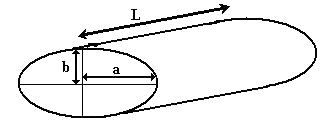# Volume calculation on a horizontal elliptical tank

The outcomes pertain to:
• a perfectly elliptical horizontal tank,
• with completely flat front and rear ends,
• which is not tilted in any way,
• which can be filled to the hilt.
In practice, many tanks have rounded or coned ends, are tilted towards the outlet, en can not be filled to the hilt, because of manholes, agitators or other outlets at the top of the tank.
Therefore the outcomes of the calculations on this page can only be used for indicative purposes. It might help you to estimate the content of a tank.

Do not use the outcomes to ascertain the commercial value of the contents of a tank. For trustworthy measuring of the liquid volume inside a particular tank, the tank must be calibrated by professionals of the local official calibration authorities.

### Total volume calculation of an elliptical tank

The calculation tool below makes it easy to determine the maximum volume of a horizontal oval-shaped tank. The "Create Dipstick Chart"-button can be used to derive a simple chart, for a quick reference to the volume of a partially filled oval tank, when the fluid depth, and the tank dimensions are known.

## Elliptical Tank Volume Calculator

All dimensions are in centimeters, volume is in litres (rounded number).
Both diameter and length must be entered & calculated first in order to create a corresponding Dipstick Chart.

 Tank DimensionsHorizontal Tank Diameter 2×a (in cm.): Vertical Tank Diameter 2×b (in cm.): Tank Length L (in cm.): Volume (in ltr.):
(use a dot ( . ) as a decimal point to compute with non-integer values)

(empty all fields)

(opens a new window)

### Volume calculation on a partially filled elliptical tank

This second tool can provide a single detailed value of the volume of a partially filled horizontal oval tank. Just insert the horizontal tank diameter, the vertical tank diameter, the tank length, and the reading on the dip stick, ruler, or measuring tape.
For example, if the horizontal tank diameter is 110,5cm, the vertical tank diameter is 80,3cm, the tank length is 350,8cm, and the liquid depth is 69,1cm, then the corresponding liquid volume equals 2237,8 ltr.

## Elliptical Tank Level Calculator

All dimensions are in centimeters, volume is in liters.
Both diameters and length must be entered as well as the reading on the dip stick.

Tank Dimensions Liquid level as read on the dip stickHorizontal Tank Diameter 2×a (in cm.): Vertical Tank Diameter 2×b (in cm.): Tank Length L (in cm.): Liquid Level h (in cm.): Volume (in ltr.):
(use a dot ( . ) as a decimal point to compute with non-integer values)

(empty all fields)